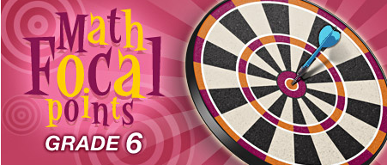### Expressions and Equations

Students write mathematical expressions and equations that correspond to given situations, they evaluate expressions, and they use expressions and formulas to solve problems. They understand that variables represent numbers whose exact values are not yet specified, and they use variables appropriately. Students understand that expressions in different forms can be equivalent, and they can rewrite an expression to represent a quantity in a different way (e.g., to make it more compact or to feature different information). Students know that the solutions of an equation are the values of the variables that make the equation true. They solve simple one-step equations by using number sense, properties of operations, and the idea of maintaining equality on both sides of an equation. They construct and analyze tables (e.g., to show quantities that are in equivalent ratios), and they use equations to describe simple relationships (such as 3x = y) shown in a table (NCTM, 2006, p. 18).

Working with variables and simple equations is the focus in these resources. Each engages middle school students in an interactive experience in which they write and evaluate expressions, work with one-step equations, or apply algebraic thinking to problems.

Late delivery This interactive math game provides exercises in substituting for variables. Players must help the mail carrier deliver letters to houses with addresses like 3(a + 2). The value of a is held by Dougal, the dog guarding the house. The algebraic expressions become more complex according to the level of difficulty selected. Tips for students are available as well as an explanation of the key ideas underlying the game.

Number tricks: bet I can guess your color! This activity opens with a cartoon in which two characters present students with directions for arriving at a special number. Students then correlate their special number with a letter of the alphabet and choose a color that begins with that letter. The characters bet that the color is yellow, and students are challenged to discover why the bet would be correct. The activity introduces algebraic procedures by demonstrating an algebraic representation in which the students' special number is added and subtracted in such a way that the operations do not affect the answer. Related questions present additional number tricks for students to solve.

Pan Balance - Expressions This interactive pan balance allows students to enter numeric or algebraic expressions. The students can "weigh" the expressions by entering them on either side of the balance and seeing if they are equivalent. The Exploration section offers teachers an interesting class activity.

Function machine (grades 6-8) Operating under a secret rule, the function machine uses numbers input by the students to generate output. Students compare the input (domain) to the output (range) to find the function rule. The analogy of a function machine is a basic, strong visual that holds up even in advanced study of functions. MSP full record

The Handshake Problem This two-lesson unit allows students to discover patterns in a fictional but real-world scenario: How many handshakes occur when the nine Supreme Court justices shake hands with each other? Students explore through a table, a graph, and finally an algebraic formula—the number of handshakes in any size group. A second pattern is explored, that of triangular numbers; again, students generalize the pattern with variables. The lessons are well illustrated and include background information for the teacher. (From Illuminations, National Council of Teachers of Mathematics Vision for School Mathematics — MSP full record)

Body mass?: what's your index? In this activity, students learn about the body mass index (BMI) formula and how it can be used to determine health risk. Essentially, this is an exercise in substituting values into an algebraic expression. The page contains links to a solution hint, the solution, and other math questions, such as how the BMI formula can be written to show weight in kilograms and height in centimeters. Students and their families are challenged to use the BMI formula to determine their health risks.

Smiles: which is worth more, a smile or a frown? This activity offers a logic problem in which students are shown an array of smiling, frowning, and neutral faces. Each row and each column add up to a different dollar amount, and students are challenged to determine how much a smile is worth. As noted on the page, "Reasoning about unknowns is essential to studying equations."

Equation Match Students find the matching pairs of equations by working out the value of x in each equation. Level 1 offers a set of one-step equations, which changes on each return to the game. This is an excellent interactive game.

Variables and Unknowns This is a PowerPoint lesson on variables, designed with the middle schooler in mind. The lesson uses color, sound, and animation to illustrate necessary concepts and procedures. Students are shown how to substitute the value of a variable in place of the variable's symbol in an algebraic expression. The concept of solving an equation for an unknown variable value is also presented.

Traffic Jam Activity The activity: there are seven stepping stones and six people, three on the left-hand stones, facing the center, and three on the right-hand stones. Everyone must move so that the people originally standing on the right exchange places with those on the left. How many moves does it take? What if there are eight people? 10? Students explore the situation through large movement experience, manipulatives, and an interactive Java applet. They look for patterns and, most importantly, write their conclusion algebraically. You’ll find the lesson plan well explained and complete.

Reaching New Heights Measuring, collecting and interpreting data, using variables—this complete lesson has it all! Students explore the relationship between two variables—height and arm span—then create a scatterplot of the data, and discuss the graph. This lesson is an excellent way to build the foundation for the study of functions. Teacher support and information about related research are included.

Non-profit Tax ID # 203478467